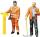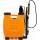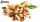Boots

Master shoemaker has three apprentices. First do one pair of boots for two days, second for 1 day, third on 1.5 day. If they worked together, for how long it would take made a couple of boots?

Result

x =  11.077 h

Solution:Leave us a comment of this math problem and its solution (i.e. if it is still somewhat unclear...):Be the first to comment!To solve this verbal math problem are needed these knowledge from mathematics:

Looking for help with calculating harmonic mean? Looking for a statistical calculator? Need help calculate sum, simplify or multiply fractions? Try our fraction calculator. Do you have a linear equation or system of equations and looking for its solution? Or do you have quadratic equation?

Next similar math problems:

1. Cleaning windowsCleaning company has to wash all the windows of the school. The first day washes one-sixth of the windows of the school, the next day three more windows than the first day and the remaining 18 windows washes on the third day. Calculate how many windows ha
2. Construction of roadFor the construction of the road dam, it is supposed to transport six trucks a total of 18 days. After three days, two more cars were sent to the construction site. How long will it take to carry the material?
3. FractionsThree-quarters of an unknown number are 4/5. What is 5/6 of this unknown number?
4. WorkersTwo workers fulfill a certain task when working together for 5 days, the first if he worked himself done work in 13 days. How many days will do whole job a second worker?
5. Working together on projectTwo employees working on the project. First make it alone for 20 days the second for 24 days. How many days will take to complete project if 5 days they work together then first will take two days holiday and after returning they completes a project toget
6. The factoryThe factory z can complete an order in 12 days, factory p in 18 days. For how many days does an order completed when the first two days work only the factory z and then both factories?
7. SprayersThe vineyard can be sprayed first sprayer in 5 hours and with the second in 8 hours. How long would it take if we use sprayers both at once?
8. Six yearsIn six years Jan will be twice as old as he was six years ago. How old is he?
9. Equation with fractionsSolve equation: ? It is equation with fractions.
10. EqnSolve equation with fractions: 2x/3-50=40+x/4
11. UN 1If we add to an unknown number his quarter, we get 210. Identify unknown number.
12. Fraction + eqSolve following simple equation with fractions: -5/6(8+5b) = 75 + 5/3b
13. Bag of peanutsJoe eat 1/3 of a bag of peanuts, mark eat 1/4 of the remaining in the bag of peanuts, Alvin eat 1/2 of the remaining bag of peanuts, peter eat 10 peanuts, there are 71 peanuts left. Hon many peanuts were in the bags?
14. PumpingThe tank is filled with a larger pump in 12 hours and with scond pump in 15 hours. For how long does the tank fill when both pumps are switched on at the same time?
15. Equation with xSolve the following equation: 2x- (8x + 1) - (x + 2) / 5 = 9
16. Unknown numberI think the number - its sixth is 3 smaller than its third.
17. Unknown numberIdentify unknown number which 1/5 is 40 greater than one tenth of that number.